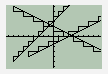Visit Henri Picciotto's Math Education Page. Send me e-mail .

STAIRA program for Texas Instruments graphing calculators
by Henri Picciotto

See the corresponding worksheets (TI-82/83/84 and TI-89).

The activity is based on "STAIR", a simple program I wrote for the TI calculators. (Thanks to Dennis Dougherty for debugging the TI-83/84 version.)

Familiarize yourself with the program by doing the activity yourself. Note that Part II requires students to already know how to use the STAT EDIT screen to enter the coordinates of individual points and (2nd) STAT PLOT graph them as "fat dots".

Preferably, download the program (TI-82/83/84, TI-89, or TI-92.) and use the TI link to get them into your calculator. If you do not have the TI link, enter the programs by hand, using the appropriate version below. Replace "->" with the "Store" arrow, "<=" with the "less than or equal" symbol, and ">=" with the "greater than or equal" symbol.

TI-82/83/84 version:

(Thanks to Dennis Dougherty for debugging this.)

Disp "STARTING POINT"
Input "X?",C:C->M
Input "Y?",D:D->N
Input "RISE? ",B
Input "RUN? ",A
While C>=Xmin and C<=Xmax and D>=Ymin and D<=Ymax
Line(C,D,C,D+B)
Line(C,D+B,C+A,D+B)
C+A->C:D+B->D
End
Line(C,D,M,N)

TI-89 version:

()
Prgm

Local usermode
getMode("all")->usermode
setGraph("Axes","ON")
setMode("Split Screen","FULL")
Đ15.8->xmin:15.8->xmax
Đ7.6->ymin:7.6->ymax
1->xscl
1->yscl

Local dostair,clean

Define dostair()=Prgm

Dialog
Title "STAIR"
Text " Starting point"
Request "x",v
Request "y",w
Text " Slope"
Request "Run",c
Request "Rise",d
EndDlog
expr(v)->x
x->m
expr(w)->y
y->n
expr(c)->a
expr(d)->b

While x>=xmin and x<=xmax and y>=ymin and y<=ymax
If a=0 and b=0:Exit
Line x,y,x,y+b
Line x,y+b,x+a,y+b
x+a->x:y+b->y
EndWhile
Line x,y,m,n

Pause

EndPrgm

Define clean()=Prgm
ClrDraw
DispG
1->ch
EndPrgm

Loop
PopUp {"Stair","Erase","Quit"},ch
If ch=1 Then:dostair()
ElseIf ch=2 Then:clean()
ElseIf ch=3 Then:setMode(usermode):Exit
EndIf
EndLoop

EndPrgm

TI-92 version:

()
Prgm

setGraph("Axes","ON")
setMode("SplitScreen","FULL")
-23.8->xmin:23.8->xmax
-10.2->ymin:10.2->ymax
1->xscl
1->yscl

Local dostair,clean

Define dostair()=Prgm

Dialog
Title "STAIR"
Text " Starting point"
Request "x",v
Request "y",w
Text " Slope"
Request "Run",c
Request "Rise",d
EndDlog

expr(v)->x
x->m
expr(w)->y
y->n
expr(c)->a
expr(d)->b

While x>=xmin and x<=xmax and y>=ymin and y<=ymax
If a=0 and b=0:Exit
Line x,y,x,y+b
Line x,y+b,x+a,y+b
x+a->x:y+b->y
EndWhile
Line x,y,m,n

Pause

EndPrgm

Define clean()=Prgm
ClrDraw
DispG
1->ch
EndPrgm

Loop
PopUp {"Stair","Erase","Quit"},ch
If ch=1 Then:dostair()
ElseIf ch=2 Then:clean()
ElseIf ch=3 Then:Exit
EndIf
EndLoop

setMode("Split 1 App","Home")
DelVar a,b,m,n,x,y,ch

EndPrgm

 Visit Henri Picciotto's Math Education Page. Send me e-mail .